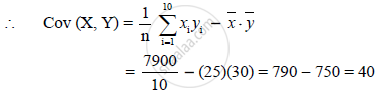HSC Arts 12th Board ExamMaharashtra State Board
Share

# In a Bivariate Data, N = 10, Bar X = 25, Bary = 30 and Sum Xy = 7900. Find Cov(X,Y) - HSC Arts 12th Board Exam - Mathematics and Statistics

ConceptStatistics Bivariate Frequency Distribution

#### Question

In a bivariate data, n = 10, bar x = 25, bary = 30 and sum xy = 7900. Find cov(X,Y)

#### Solution

n = 10, bar x = 25, bary = 30 and sum xy = 7900Is there an error in this question or solution?

#### APPEARS IN

2012-2013 (October) (with solutions)
Question 4.2.4 | 2.00 marks
Solution In a Bivariate Data, N = 10, Bar X = 25, Bary = 30 and Sum Xy = 7900. Find Cov(X,Y) Concept: Statistics - Bivariate Frequency Distribution.
S